### Calculate displacement given work and force.

#### Example question:

A force of 16 N does 120 J of work on an object. Over what displacement did this force work?

#### Given:

force: F = 16 N

work: W = 120 J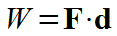Starting formula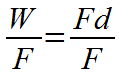Algebra: Divide each side by F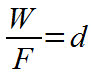Algebra: Cancel F's on right side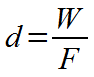Algebra: Switch left and right sides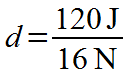Plug in numbers with units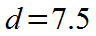Divide: 120 / 16 = 7.5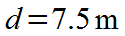Final result with significant figures and unit